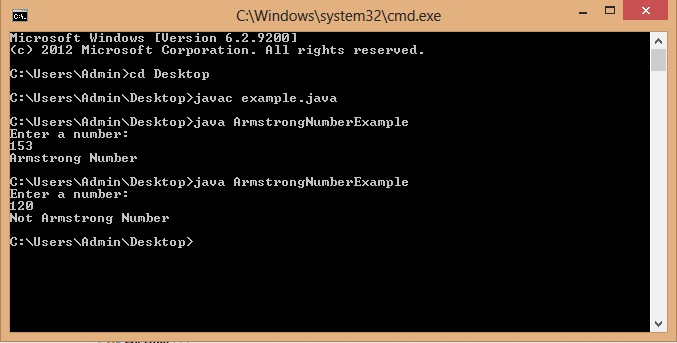# Program for Armstrong Number in Java

Armstrong number is a n digit number such that the sum of digits raised to the power n is equal to the number.

For example:

153 is armstrong number because here n=3 and 13+53+33=153.

120 is not armstrong number because 13+23+03!=120.

Below I have shared a Java program that checks whether a number is armstrong number or not.

## Program for Armstrong Number in Java

Output### 2 thoughts on “Program for Armstrong Number in Java”

1.An Armstrong number of three digits is an integer such that the sum of the cubes of its digits is equal to the number itself.
For example, 371 is an Armstrong number since 3**3 + 7**3 + 1**3 = 371.

2.best programming site i have ever used